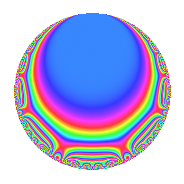# Properties

 Label 4020.2.a.cLevel 4020 Weight 2 Character orbit 4020.a Self dual Yes Analytic conductor 32.100 Analytic rank 1 Dimension 4 CM No Inner twists 1

# Learn more about

## Newspace parameters

 Level: $$N$$ = $$4020 = 2^{2} \cdot 3 \cdot 5 \cdot 67$$ Weight: $$k$$ = $$2$$ Character orbit: $$[\chi]$$ = 4020.a (trivial)

## Newform invariants

 Self dual: Yes Analytic conductor: $$32.0998616126$$ Analytic rank: $$1$$ Dimension: $$4$$ Coefficient field: 4.4.98117.1 Coefficient ring: $$\Z[a_1, \ldots, a_{7}]$$ Coefficient ring index: $$1$$ Fricke sign: $$1$$ Sato-Tate group: $\mathrm{SU}(2)$

## $q$-expansion

Coefficients of the $$q$$-expansion are expressed in terms of a basis $$1,\beta_1,\beta_2,\beta_3$$ for the coefficient ring described below. We also show the integral $$q$$-expansion of the trace form.

 $$f(q)$$ $$=$$ $$q$$ $$+ q^{3}$$ $$- q^{5}$$ $$+ \beta_{1} q^{7}$$ $$+ q^{9}$$ $$+O(q^{10})$$ $$q$$ $$+ q^{3}$$ $$- q^{5}$$ $$+ \beta_{1} q^{7}$$ $$+ q^{9}$$ $$+ ( -1 - \beta_{1} + \beta_{3} ) q^{11}$$ $$+ ( -1 - \beta_{2} ) q^{13}$$ $$- q^{15}$$ $$+ ( -2 - \beta_{1} + \beta_{2} - \beta_{3} ) q^{17}$$ $$+ ( 1 + \beta_{2} - \beta_{3} ) q^{19}$$ $$+ \beta_{1} q^{21}$$ $$-3 q^{23}$$ $$+ q^{25}$$ $$+ q^{27}$$ $$+ ( -1 - \beta_{1} ) q^{29}$$ $$+ ( -\beta_{2} - \beta_{3} ) q^{31}$$ $$+ ( -1 - \beta_{1} + \beta_{3} ) q^{33}$$ $$-\beta_{1} q^{35}$$ $$+ ( 3 + 2 \beta_{1} ) q^{37}$$ $$+ ( -1 - \beta_{2} ) q^{39}$$ $$+ ( -4 - \beta_{2} + \beta_{3} ) q^{41}$$ $$+ ( -2 - \beta_{2} + 2 \beta_{3} ) q^{43}$$ $$- q^{45}$$ $$+ ( -1 - 4 \beta_{1} + \beta_{2} - 2 \beta_{3} ) q^{47}$$ $$+ ( -3 + \beta_{2} - \beta_{3} ) q^{49}$$ $$+ ( -2 - \beta_{1} + \beta_{2} - \beta_{3} ) q^{51}$$ $$+ ( -1 + \beta_{2} + \beta_{3} ) q^{53}$$ $$+ ( 1 + \beta_{1} - \beta_{3} ) q^{55}$$ $$+ ( 1 + \beta_{2} - \beta_{3} ) q^{57}$$ $$+ ( -7 + 4 \beta_{1} + \beta_{2} ) q^{59}$$ $$+ ( -7 + \beta_{2} - \beta_{3} ) q^{61}$$ $$+ \beta_{1} q^{63}$$ $$+ ( 1 + \beta_{2} ) q^{65}$$ $$- q^{67}$$ $$-3 q^{69}$$ $$+ ( -5 + 2 \beta_{1} - 2 \beta_{2} + \beta_{3} ) q^{71}$$ $$+ ( -3 \beta_{1} + \beta_{2} + \beta_{3} ) q^{73}$$ $$+ q^{75}$$ $$+ ( -5 - 2 \beta_{1} - \beta_{2} + \beta_{3} ) q^{77}$$ $$+ ( -4 - \beta_{2} ) q^{79}$$ $$+ q^{81}$$ $$+ ( 3 - 2 \beta_{1} - 3 \beta_{3} ) q^{83}$$ $$+ ( 2 + \beta_{1} - \beta_{2} + \beta_{3} ) q^{85}$$ $$+ ( -1 - \beta_{1} ) q^{87}$$ $$+ ( -5 + 2 \beta_{1} + 5 \beta_{3} ) q^{89}$$ $$+ ( -4 \beta_{1} - \beta_{2} ) q^{91}$$ $$+ ( -\beta_{2} - \beta_{3} ) q^{93}$$ $$+ ( -1 - \beta_{2} + \beta_{3} ) q^{95}$$ $$+ ( -4 + 5 \beta_{1} + 3 \beta_{3} ) q^{97}$$ $$+ ( -1 - \beta_{1} + \beta_{3} ) q^{99}$$ $$+O(q^{100})$$ $$\operatorname{Tr}(f)(q)$$ $$=$$ $$4q$$ $$\mathstrut +\mathstrut 4q^{3}$$ $$\mathstrut -\mathstrut 4q^{5}$$ $$\mathstrut +\mathstrut q^{7}$$ $$\mathstrut +\mathstrut 4q^{9}$$ $$\mathstrut +\mathstrut O(q^{10})$$ $$4q$$ $$\mathstrut +\mathstrut 4q^{3}$$ $$\mathstrut -\mathstrut 4q^{5}$$ $$\mathstrut +\mathstrut q^{7}$$ $$\mathstrut +\mathstrut 4q^{9}$$ $$\mathstrut -\mathstrut 5q^{11}$$ $$\mathstrut -\mathstrut 5q^{13}$$ $$\mathstrut -\mathstrut 4q^{15}$$ $$\mathstrut -\mathstrut 8q^{17}$$ $$\mathstrut +\mathstrut 5q^{19}$$ $$\mathstrut +\mathstrut q^{21}$$ $$\mathstrut -\mathstrut 12q^{23}$$ $$\mathstrut +\mathstrut 4q^{25}$$ $$\mathstrut +\mathstrut 4q^{27}$$ $$\mathstrut -\mathstrut 5q^{29}$$ $$\mathstrut -\mathstrut q^{31}$$ $$\mathstrut -\mathstrut 5q^{33}$$ $$\mathstrut -\mathstrut q^{35}$$ $$\mathstrut +\mathstrut 14q^{37}$$ $$\mathstrut -\mathstrut 5q^{39}$$ $$\mathstrut -\mathstrut 17q^{41}$$ $$\mathstrut -\mathstrut 9q^{43}$$ $$\mathstrut -\mathstrut 4q^{45}$$ $$\mathstrut -\mathstrut 7q^{47}$$ $$\mathstrut -\mathstrut 11q^{49}$$ $$\mathstrut -\mathstrut 8q^{51}$$ $$\mathstrut -\mathstrut 3q^{53}$$ $$\mathstrut +\mathstrut 5q^{55}$$ $$\mathstrut +\mathstrut 5q^{57}$$ $$\mathstrut -\mathstrut 23q^{59}$$ $$\mathstrut -\mathstrut 27q^{61}$$ $$\mathstrut +\mathstrut q^{63}$$ $$\mathstrut +\mathstrut 5q^{65}$$ $$\mathstrut -\mathstrut 4q^{67}$$ $$\mathstrut -\mathstrut 12q^{69}$$ $$\mathstrut -\mathstrut 20q^{71}$$ $$\mathstrut -\mathstrut 2q^{73}$$ $$\mathstrut +\mathstrut 4q^{75}$$ $$\mathstrut -\mathstrut 23q^{77}$$ $$\mathstrut -\mathstrut 17q^{79}$$ $$\mathstrut +\mathstrut 4q^{81}$$ $$\mathstrut +\mathstrut 10q^{83}$$ $$\mathstrut +\mathstrut 8q^{85}$$ $$\mathstrut -\mathstrut 5q^{87}$$ $$\mathstrut -\mathstrut 18q^{89}$$ $$\mathstrut -\mathstrut 5q^{91}$$ $$\mathstrut -\mathstrut q^{93}$$ $$\mathstrut -\mathstrut 5q^{95}$$ $$\mathstrut -\mathstrut 11q^{97}$$ $$\mathstrut -\mathstrut 5q^{99}$$ $$\mathstrut +\mathstrut O(q^{100})$$

Basis of coefficient ring in terms of a root $$\nu$$ of $$x^{4}\mathstrut -\mathstrut$$ $$x^{3}\mathstrut -\mathstrut$$ $$8$$ $$x^{2}\mathstrut +\mathstrut$$ $$4$$ $$x\mathstrut +\mathstrut$$ $$1$$:

 $$\beta_{0}$$ $$=$$ $$1$$ $$\beta_{1}$$ $$=$$ $$\nu$$ $$\beta_{2}$$ $$=$$ $$\nu^{3} - 8 \nu - 1$$ $$\beta_{3}$$ $$=$$ $$\nu^{3} - \nu^{2} - 8 \nu + 3$$
 $$1$$ $$=$$ $$\beta_0$$ $$\nu$$ $$=$$ $$\beta_{1}$$ $$\nu^{2}$$ $$=$$ $$-$$$$\beta_{3}\mathstrut +\mathstrut$$ $$\beta_{2}\mathstrut +\mathstrut$$ $$4$$ $$\nu^{3}$$ $$=$$ $$\beta_{2}\mathstrut +\mathstrut$$ $$8$$ $$\beta_{1}\mathstrut +\mathstrut$$ $$1$$

## Embeddings

For each embedding $$\iota_m$$ of the coefficient field, the values $$\iota_m(a_n)$$ are shown below.

For more information on an embedded modular form you can click on its label.

Label $$\iota_m(\nu)$$ $$a_{2}$$ $$a_{3}$$ $$a_{4}$$ $$a_{5}$$ $$a_{6}$$ $$a_{7}$$ $$a_{8}$$ $$a_{9}$$ $$a_{10}$$
1.1
 −2.60457 −0.184077 0.668475 3.12017
0 1.00000 0 −1.00000 0 −2.60457 0 1.00000 0
1.2 0 1.00000 0 −1.00000 0 −0.184077 0 1.00000 0
1.3 0 1.00000 0 −1.00000 0 0.668475 0 1.00000 0
1.4 0 1.00000 0 −1.00000 0 3.12017 0 1.00000 0
 $$n$$: e.g. 2-40 or 990-1000 Significant digits: Format: Complex embeddings Normalized embeddings Satake parameters Satake angles

## Inner twists

This newform does not admit any (nontrivial) inner twists.

## Atkin-Lehner signs

$$p$$ Sign
$$2$$ $$-1$$
$$3$$ $$-1$$
$$5$$ $$1$$
$$67$$ $$1$$

## Hecke kernels

This newform can be constructed as the kernel of the linear operator $$T_{7}^{4}$$ $$\mathstrut -\mathstrut T_{7}^{3}$$ $$\mathstrut -\mathstrut 8 T_{7}^{2}$$ $$\mathstrut +\mathstrut 4 T_{7}$$ $$\mathstrut +\mathstrut 1$$ acting on $$S_{2}^{\mathrm{new}}(\Gamma_0(4020))$$.